### Software and R packages installation and working directory setup.

• Software:

• Netlogo (for Problem 1)

• R (for Problem 2)

• R packages:

• knitr, shiny, Matrix, deSolve, EpiModel, ggplot2

• To install these packages, just type the following command into the RStudio or R console to install all of the packages: install.packages (c("shiny","knitr","Matrix","deSolve","EpiModel","ggplot2"))

• You can also click Tools > Install Packages in RStudio, and then key in the package names. The packages will be automatically downloaded.

• Set working directory to the location where you’ve saved the workshop files:

• use setwd("the_path") (eg. setwd("~/Desktop/iTiMS_workshop"))
• For people who use Rstudio, you can also navigate to your working directory from the Files panel at the right-hand site, and then click More > Set as Working Dirctory

### Tips for using the exercise notebook.

The files we will need for this exercise are listed below. All files can be downloaded here (some are in the folder “AdditionalExercises”).

File name Content
itims_workshop.Rmd The exercise note in R markdown
itims_workshop.html The html version of the exercise note (can be viewed in web browsers)
model_shiny.Rmd shiny interface for the models we use in the exercise
empty_example_model.nlogo the empty example model used in Problem 1C
SIR-agent-based.nlogo An agent based model of disease transmission in NetLogo
SIR-deterministic.nlogo A deterministic population model of disease transmission in NetLogo
SIR-stochastic.nlogo A stochastic population model of disease transmission in NetLogo
turtle_color.nlogo An example model from optional exercises

This notebook is written in R Markdown (the .Rmd file). This generates the HTML version of the notebook (the .html file), which can be viewed in your browser. To run the R code in the notebook:

1. You can copy the code in R code chunks from the .html file (you can also copy from the .Rmd if you prefer), paste it into the RStudio or R console, and hit “enter”.

2. If you don’t want to have to keep copy-pasting into the console and you are using RStudio, you can also make a new script file and paste the R code chunks into that—then you can execute those lines of code by highlighting them and then clicking the drop-down arrow of Run button and then Run Selected Line(s), or just hit ctrl-enter (windows) or cmd-return (mac) to run the highlighted code.

3. Alternatively, if you are using RStudio (all versions), you can highlight the code you want to excute in the .Rmd file and then click the drop-down arrow of Run button and click Run Selected Line(s). You can also just hit ctrl-enter (windows) or cmd-return (mac) to run the highlighted code.

4. If your version of RSutdio is 1.0 or higher (RStudio > About RStudio), you can also click the right arrow at the upper right hand corner of a R code chunk to excute the chunk. You will see the outputs immediately after the R code chunck. In order to see the outputs in the Viewer or Plots window, click the gear icon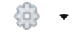and then check Chunk Output in Console.

Note1: in order to create a script to save the R code you work on in RStudio, you can click the New File icon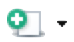and then select R Script. To run the whole script, just click the Run button.

Note2: the itims_workshop.html can be generated from itims_workshop.Rmd by clicking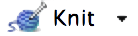If you want to record your answers to the problems as you go, the easiest way is probably to use this form (plus, that way we can see how people are progressing and what you all discover!). But if you’d rather not, feel free to write your answers on paper or in the text boxes below. Just be careful—answers in the text boxes below will not be saved if you reload the page!

### Problem 1A: Simple rules can lead to complex behavior.

During the 1960s the economist Thomas Schelling researched neighborhood racial segregation and residential preferences. Using a simple model, he showed that individuals can have only mild preferences for the same type and yet these subtle preferences can lead to societal segregation. Here, we’ll explore this with a hypothetical setting, looking at interactions between red and green turtles.

In this model, the red turtles and green turtles get along with one another. But each turtle wants to make sure that it lives near some of “its own.” That is, each red turtle wants to live next to at least some red turtles, and each green turtle wants to live next to at least some green turtles.

So, each turtle is happy if at least a certain percentage of their neighbors are the same type as them (this percent is given by the parameter %-similar-wanted in the NetLogo file). If a turtle is happy, they stay in their current location. If they are unhappy, they move to a randomly chosen new location. This continues until all the people are happy (or possibly forever).

• Let’s check out the Schelling’s Segregation Model in NetLogo (if you are using v6.0.1, please open NetLogo instead of NetLogo 3D)!

• Open NetLogo, then open File > Models Library > Sample Models > Social Science > Segregation

• Before running the simulation, click Info to read more details about the model, and click Code to see how the model is being built.

• Returning to Interface, you can click setup botton and then go botton to start the simulation. Click go again to stop the simulation. You can use the slider bar on the top panel of the window to adjust the speed of simulation. You can also right click go > Edit > unclick Forever > OK (or just click go once in v6.0.1) to see the simulation at each time step.

• By default, the turtles only need 30% of their neighbors to be the same color as them. What do you think will happen? How do you think the turtles will distribute themselves—will they cluster by turtle color or mix together?

• Start by running the model using the default settings—does what happen match what you expected?

• Next, let’s adjust the model parameters and see what happens—start by changing the %-similar-wanted. How does this alter the turtle behavior?

• Lastly adjust the population density as well as %-similar-wanted. How does population density change the results you found above?

### Problem 1B: Disease spread on networks.

Next, we’ll look at how disease spreads on contact networks between individuals. Open the Virus on a Network model, in File > Models Library > Sample Models > Networks > Virus on a Network. This model simulates disease spread model on a network, where infected individuals can either return to the susceptible class or become immune. Simulate the model a several times for a range of parameter values (We just want you to explore a bit).

• What do you notice about how the network structure affects the dynamics of the epidemic? Do some levels of connectivity or numbers of people seem to be more likely to allow an outbreak than others?

• Consider the parameters for virus-spread-chance, recovery-chance, and gain-resistance-chance. How would you expect these parameters to change the outcome of the disease outbreak?

• Adjust the three parameters in the previous question to a range of values—how does the model behavior change? Does it match what you expected?

### Problem 1C: Coding a very simple model.

Next, we will model an extremely simple system. Suppose we have a set of agents (called ‘turtles’ in NetLogo) which obey four simple rules at each time step:

• Direction: change their direction within ±20° of their current direction.
• Movement: move forward by 10 steps.
• Chameleon: if the agents are on a patch that is non-empty (i.e. not black), they change their color to match the patch
• Paint: if they are on a patch that is empty (black), they paint the patch with their color.

The code for each rule is given below. Open the empty_example_model.nlogo provided, click on Code , and then modify it by adding the rule code provided below to the “go” function so that the turtles follow each of the four rules at each time step (check the comments in the empty_example_mode.nlogo for more details). Now run the model several times. What patterns do you see?

Note: if your NetLogo is not v6.0.1, there might be warning messages when you open the file. Please just hit Continue to go on.

Rule code:

• Direction: rt random 20
• Movement: fd step-size
• Chameleon: if (pcolor != black) [set color pcolor]
• Paint: if (pcolor = black) [set pcolor color]

Change the number of steps the turtles move forward by at each time step (start with a step size of 1, then try increasing it). How do the patterns change as you increase the step size?

### Problem 2A: Compartmental models of contagion.

Next, we’ll develop and simulate a model of contagion. The model diagram and equations are: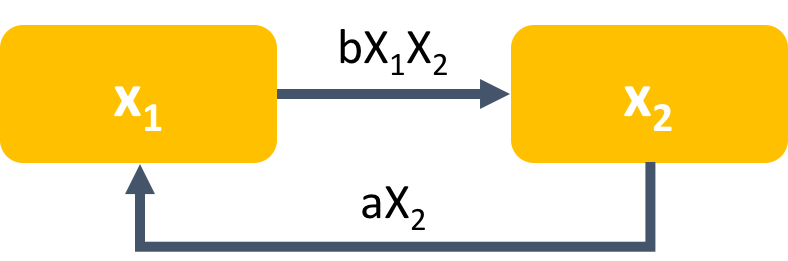$\frac{dX_1}{dt} = -bX_1X_2+aX_2$ $\frac{dX_2}{dt} = bX_1X_2-aX_2$
We can use the same model to represent three different scenarios—choose one to use for this exercise:

1. Infectious Disease Spread. In this scenario, $$X_1$$ represents the number of susceptible individuals in the population, and $$X_2$$ represents the number of infected individuals in the population. The term $$bX_1X_2$$ represents the process by which susceptible become infected, and the $$aX_2$$ term represents recovery of infected individuals (once recovered, individuals go back to the susceptible state).

2. Tobacco Use. In this scenario, $$X_1$$ represents the number of non-smoker individuals in the population, and $$X_2$$ represents the number of smokers in the population. The term $$bX_1X_2$$ represents the process by which non-smokers begin smoking, assuming that they begin smoking via peer pressure from smokers. The $$aX_2$$ term represents smokers quitting smoking.

3. Prion Conformational Changes. Prions are malformed proteins which can cause a variety of brain diseases. In this scenario, $$X_1$$ represents the number of normal prion proteins in the tissue, and $$X_2$$ represents the number of prions (malformed proteins) in the tissue. The term $$bX_1X_2$$ represents the process by which normal proteins become malformed after interacting with a prion (malformed protein). We suppose that for this prion disease there is also a treatment which returns the proteins back to their normal state at rate $$aX_2$$.

Implement and run the model in R, using either the R code below or the Shiny app (open model_shiny.Rmd, and click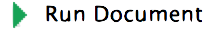), which uses an interface similar to NetLogo (i.e. you can use slider bars to change the value of parameters). What behavior do you observe? Is this what you would expect to happen for the scenario you chose?

R code :

library(deSolve)
## model equations
model1 <- function(t, x, params){
x1 = x
x2 = x

b = params
a = params

dx1 = -b*x1*x2+a*x2
dx2 = b*x1*x2-a*x2

list(c(dx1, dx2))
}
## set up initial conditions and parameter values
ini_md1 <- c(x1=99, x2=1)
param_md1 <- c(b=0.01, a=0.1)

## time steps
times<- seq(0,20,by=1)

## model simulation
res_md1<- as.data.frame(ode(y=ini_md1, times=times, func=model1, parms=param_md1, method="ode45"))
#res_md1 #print out the results from each time step

## plot epidemic curve
matplot(res_md1$time, as.data.frame(c(res_md1,res_md1)), type='l', xlab='time', ylab='counts', lwd=3, bty='l', col=c(1,3), cex.lab=1.3, cex.axis=1.1, ylim=c(0, 130)) legend("top",c("x1", "x2"), col=c(1,3),bty="n", horiz=TRUE, lwd=2, lty=1:3,cex=1.2 )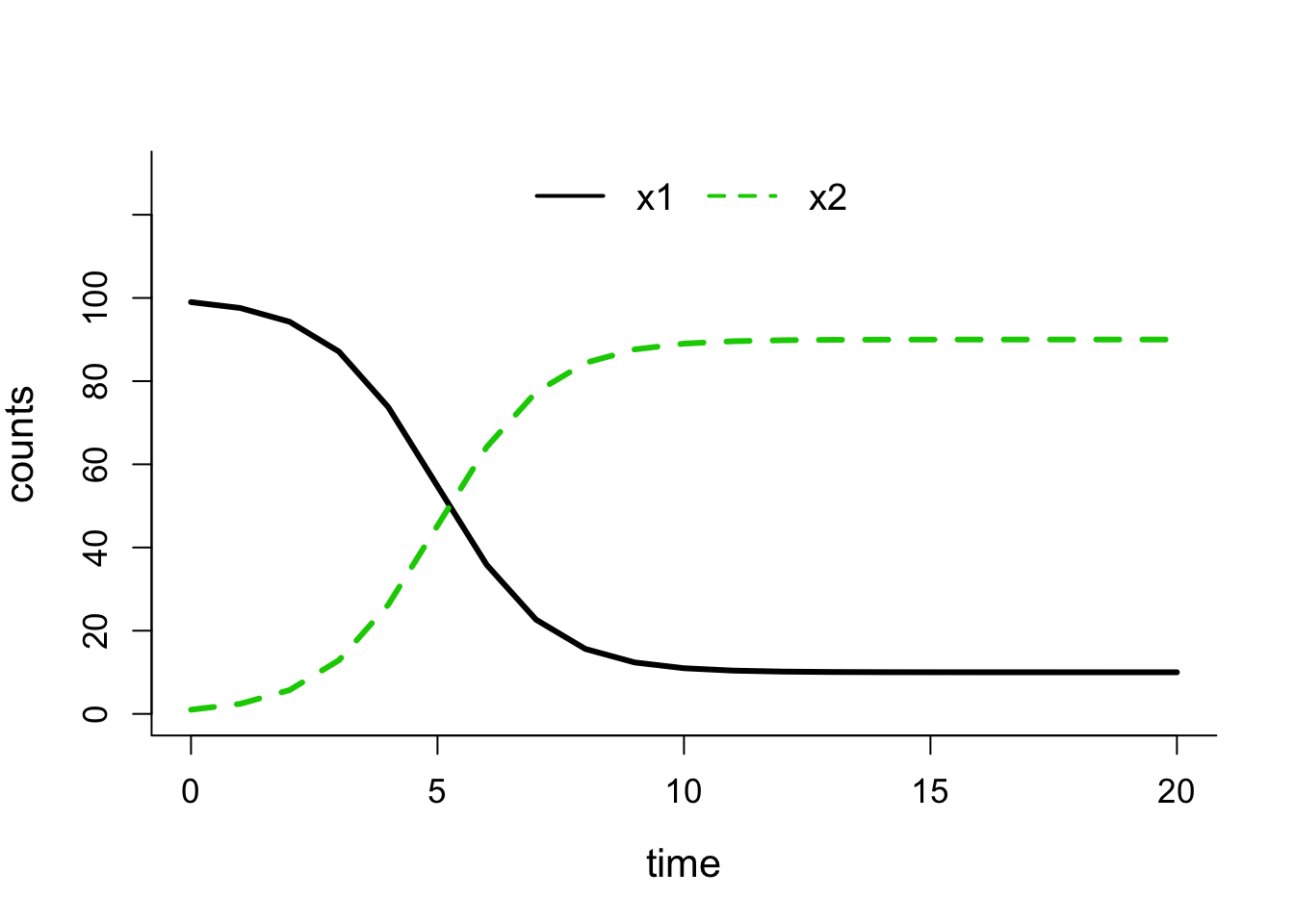### Problem 2B: Parameters and interpretation. Using the model from Problem 2A, choose one parameter (a or b). Without running the model, predict how you would expect the model behavior to change if you increased that parameter by a factor of 5, and write down your prediction. Now, change the parameter and simulate the model—does it match your prediction? Try this for the other parameter as well. ### Problem 2C: Distinguishing alternative mechanisms. Now, suppose we have two other candidate models which we might use: (R code is provided below, or you can use the same Shiny app file) • Alternate Model 1: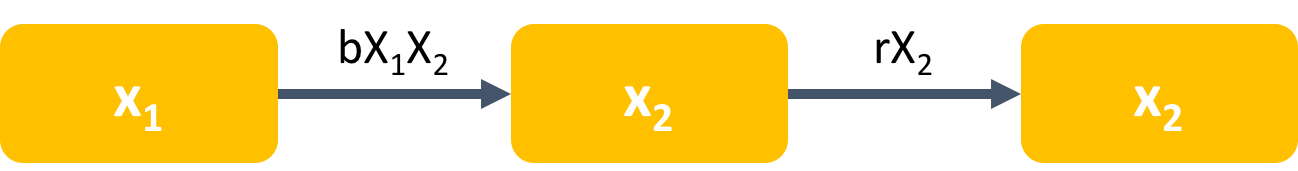$\frac{dX_1}{dt} = -bX_1X_2$ $\frac{dX_2}{dt} = bX_1X_2-rX_2$ $\frac{dX_3}{dt} =rX_2$ • Alternate Model 2: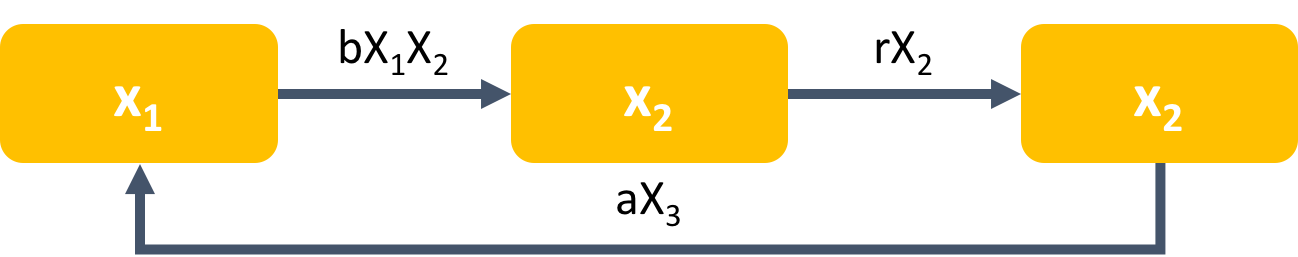$\frac{dX_1}{dt} = -bX_1X_2+aX_3$ $\frac{dX_2}{dt} = bX_1X_2-rX_2$ $\frac{dX_3}{dt} =rX_2-aX_3$ The alternate models above add to the model from Problem 2A, supposing that after $$X_2$$ (infectious/smoker/prion), a person/molecule moves to a third state, $$X_3$$. 1. For Scenario 1, $$X_3$$ represents the number of individuals who have immunity to the disease. In Alternate Model 2, they lose immunity and then return to being susceptible to the disease. 2. For Scenario 2, $$X_3$$ represents quit individuals who are resistant to smoking. We suppose that after quitting, an individual will have some resistance to re-starting smoking. In Alternate Model 2, after a while people in $$X_3$$ lose their resistance and go back to being ordinary non-smokers ($$X_1$$). 3. For Scenario 3, $$X_3$$ represents prions which have been treated. In Alternate Model 2, after some time, they return to the normal protein state ($$X_1$$). • In Alternate Models 1 and 2, what does the rate $$rX_2$$ represent? In Alternate Model 2, what does the rate $$aX_3$$ represent? R Code for both models: Alternate Model 1 ## Alternate Model 1 - equations model2 <- function(t, x, params){ x1 = x x2 = x x3 = x b = params r = params dx1 = -b*x1*x2 dx2 = b*x1*x2-r*x2 dx3 = r*x2 list(c(dx1, dx2, dx3)) } ## set up initial conditions and parameter values ini_md2 <- c(x1=99, x2=1, x3=0.0) param_md2 <- c(b=0.01, r=0.02) ## time steps times<- seq(0,20,by=1) ## model simulation res_md2<- as.data.frame(ode(y=ini_md2, times=times, func=model2, parms=param_md2, method="ode45")) #res_md2 #print out the results from each time step To plot your results: ## plot epidemic curve matplot(res_md2$time, as.data.frame(c(res_md2,res_md2,res_md2)), type='l', xlab='time', ylab='counts', lwd=3, bty='l', col=c(1,3,4), cex.lab=1.3, cex.axis=1.1, ylim=c(0, 130))
legend("top",c("x1", "x2", "x3"), col=c(1,3,4),bty="n", horiz=TRUE, lwd=2, lty=1:4,cex=1.2 )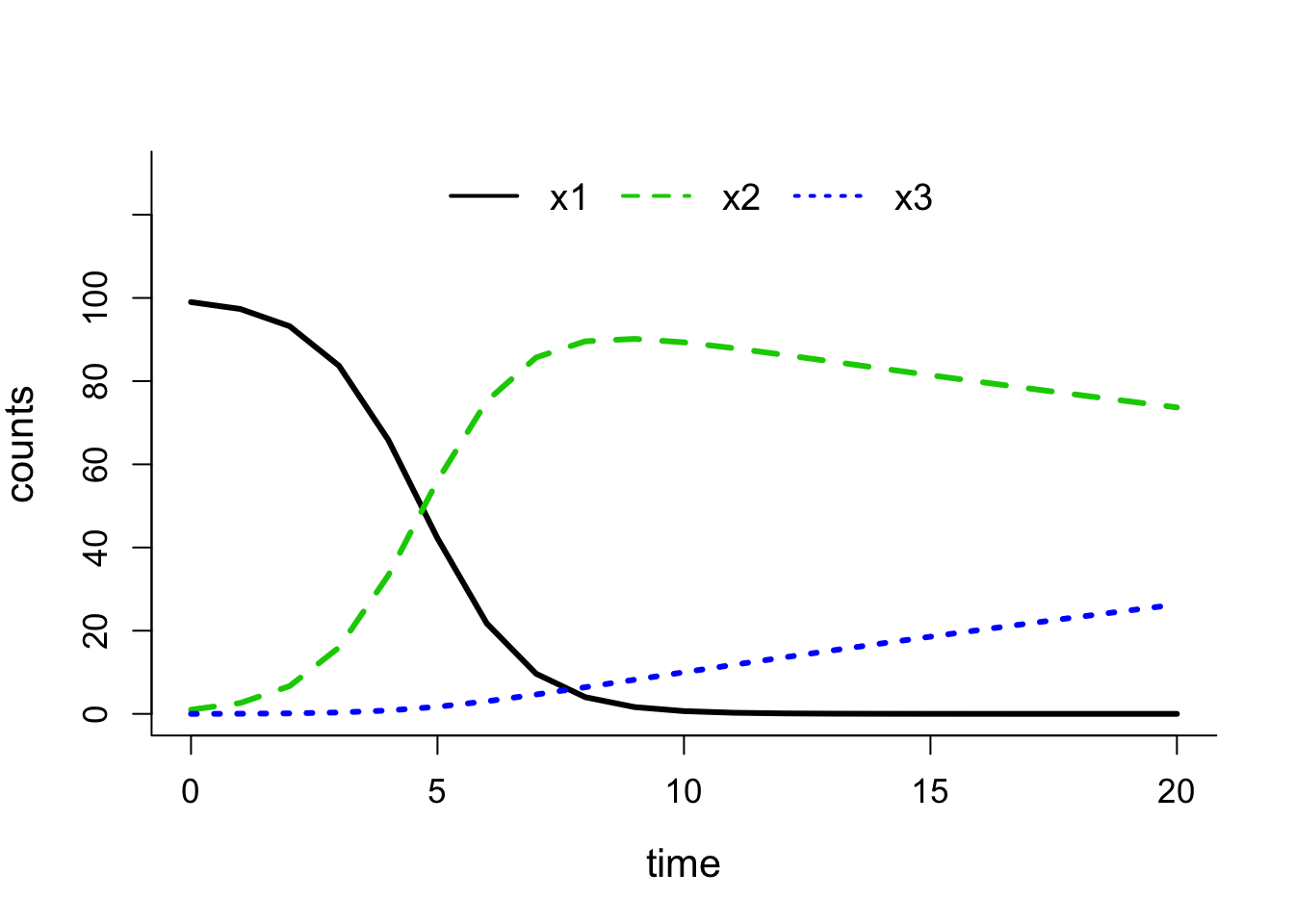Alternate Model 2

## Alternate Model 2 - equations
model3 <- function(t, x, params){
x1 = x
x2 = x
x3 = x

b = params
a = params
r = params

dx1 = -b*x1*x2+a*x3
dx2 = b*x1*x2-r*x2
dx3 = r*x2-a*x3

list(c(dx1, dx2, dx3))
}
## set up initial conditions and parameter values
ini_md3 <- c(x1=99, x2=1, x3=0.0)
param_md3 <- c(b=0.01, a=0.01, r=0.02)

## time steps
times<- seq(0,20,by=1)

## model simulation
res_md3<- as.data.frame(ode(y=ini_md3, times=times, func=model3, parms=param_md3, method="ode45"))
#res_md3 #print out the results from each time step

## plot epidemic curve
legend("top",c("x1", "x2", "x3"), col=c(1,3,4),bty="n", horiz=TRUE, lwd=2, lty=1:4,cex=1.2 )